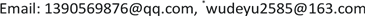Copyright © 2019 by authors and Hans Publishers Inc.1. 引言

Hilbert空间中线性算子数值域是二次型和Rayleigh商逻辑上的推广，它具有深厚的理论基础和广泛的应用价值。Hilbert空间中有界线性算子 A 的数值域定义为

W ( A ) = { ( A x , x ) ( x , x ) ： ( x , x ) ≠ 0 } ,

σ p ( A ) ∪ ​ σ r ( A ) ⊂ W ( A ) , σ ( A ) ⊂ W ( A ) ¯ .

W 2 ( T ) = { λ ∈ C : ∃ f , g ≠ 0 使 得 | ( A f , f ) ‖ f ‖ 2 − λ ( B g , f ) ‖ f ‖ ‖ g ‖ ( C f , g ) ‖ f ‖ ‖ g ‖ ( D g , g ) ‖ g ‖ 2 − λ | = 0 } 。

w 2 ( T ) = sup { | λ | : λ ∈ W 2 ( T ) } 。

r ( T ) ≤ w 2 ( T ) ≤ w ( T ) ≤ ‖ T ‖ 。

w ( T S ) ≤ 4 w ( T ) w ( S ) 。

w ( T S ) ≤ 2 w ( T ) w ( S ) 。

w ( T S ) ≤ w ( T ) w ( S ) 。

w ( T n ) ≤ [ w ( T ) ] n , n = 1 , 2 , 3 , ⋯

2. 预备知识

w ( T S ) ≤ 4 w ( T ) w ( S ) ；

w ( T S ) ≤ 2 w ( T ) w ( S ) 。

w ( T S ) ≤ w ( T ) w ( S ) 。

w ( T n ) ≤ [ w ( T ) ] n

w 2 ( T ) = max { w ( A ) , w ( D ) } 。

W 2 ( T ) = W ( A ) ∪ W ( D ) 。

3. 主要结果及其证明

w ( T S ) ≤ 4 w ( T ) w (S)

w 2 ( T S ) ≤ 4 w 2 ( T ) w 2 (S)

T = [ 0 1 0 0 ] , S = [ 0 0 1 0 ] ，

w 2 ( T S ) ≤ 4 w 2 ( T ) w 2 ( S ) 。

w ( T S ) ≤ 4 w ( T ) w ( S ) ,

r ( T ) ≤ w 2 ( T ) ≤ w ( T ) ≤ ‖ T ‖ , r ( S ) ≤ w 2 ( S ) ≤ w ( S ) ≤ ‖ S ‖ .

w 2 ( T S ) ≤ 4 w ( T ) w (S)

r ( T ) = w ( T ) , r ( S ) = w (S)

w 2 ( T ) = w ( T ) , w 2 ( S ) = w (S)

w 2 ( T S ) ≤ 4 w 2 ( T ) w 2 (S)

w 2 ( T ) = w ( T ) , w 2 ( S ) = w ( S ) 。

w 2 ( T S ) ≤ 2 w 2 ( T ) w 2 ( S ) 。

w 2 ( T S ) ≤ 2 w 2 ( T ) w 2 ( S ) 。

w 2 ( T S ) ≤ w 2 ( T ) w 2 ( S ) 。

w ( T S ) ≤ w ( T ) w ( S ) ，

w 2 ( T S ) ≤ w ( T ) w ( S ) 。